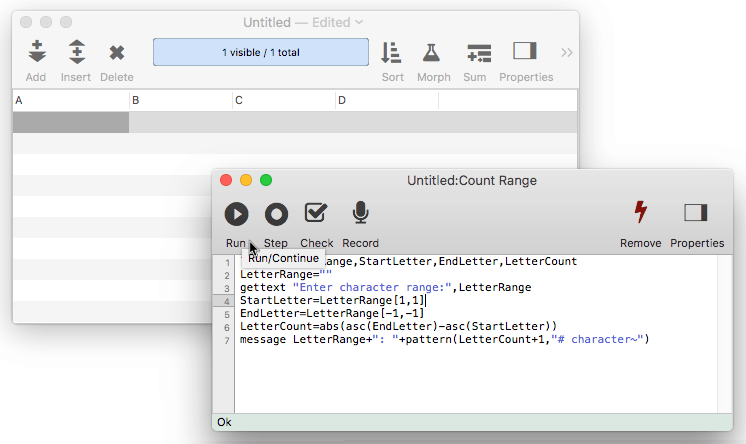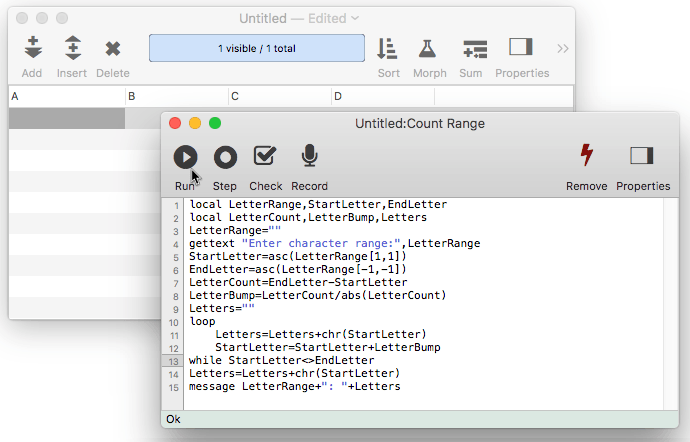Just as molecules are built from atoms, text is built from characters. And like an atom which can be divided into electrons, protons, and neutrons (among others), characters also have an internal structure. Just as with atoms, the internal structure of characters can usually be ignored, and you may want to skip the following section if you are a beginner. Sometimes however, knowledge of the internal structure of characters can be very helpful.

On modern computer systems there are over 65,000 possible characters. Each character has a number from 0 to 65,535. For example, the symbol for the letter A is represented by character number 65. The number value for each character is defined by an international standard called Unicode.

Panorama uses the Unicode values of characters when it compares two text items to see which is larger or smaller. Since the Unicode value of B (66) is greater than the Unicode value of A (65), the text item B is “larger” than A. However, the Unicode value of a (97) is greater than B (66), so the text item a is “larger” than B. You have to watch out for this problem whenever you compare text that is a mixture of upper and lower case.

#### Working with Character Values

Usually it’s not necessary to worry about the numeric value of a particular character—you can just think of it as a character. However, if you want to perform any kind of math on the character itself it is necessary to convert the character in to a number. For example you can add one to a character value to get the next character value (A ➛ B ➛ C etc.). Or you can calculate the number of characters between two characters.

Panorama has two special functions that allow you to work with character values directly. The asc( function converts a character to its Unicode numeric value. The chr( function converts an Unicode numeric value to the corresponding character.

The following example procedure asks the user to enter a range of letters, for example A-F. It uses the asc( function to convert the characters into the corresponding Unicode numeric values, then calculates the number of characters in the range.

``````local LetterRange,StartLetter,EndLetter,LetterCount
LetterRange=""
gettext "Enter character range:",LetterRange
StartLetter=LetterRange[1,1]
EndLetter=LetterRange[-1,-1]
LetterCount=abs(asc(EndLetter)-asc(StartLetter))
message LetterRange+": "+pattern(LetterCount+1,"# character~")
``````

If the person enters A-F the procedure will display A-F: 6 characters.The next example procedure is similar but actually displays a list of the characters in the range. It uses the chr( function to convert the numbers back into characters.

``````local LetterRange,StartLetter,EndLetter
local LetterCount,LetterBump,Letters
LetterRange=""
gettext "Enter character range:",LetterRange
StartLetter=asc(LetterRange[1,1])
EndLetter=asc(LetterRange[-1,-1])
LetterCount=EndLetter-StartLetter
LetterBump=LetterCount/abs(LetterCount)
Letters=""
loop
Letters=Letters+chr(StartLetter)
StartLetter=StartLetter+LetterBump
while StartLetter<>EndLetter
Letters=Letters+chr(StartLetter)
message LetterRange+": "+Letters
``````

If the person enters A-F the procedure will display A-F: ABCDEF. If the person enters Z-U the procedure will display Z-U: ZYXWVU.Warning: Don’t confuse the asc( and chr( functions with the val( and str( functions. The asc( and chr( functions convert single characters based on their ASCII values. The val( and str( functions convert entire text items based on the number the characters spell out. For example asc(“4”) is 52, because 52 is the Unicode value of the character “4.” On the other hand, val(“4”) is 4. Confused? In most ordinary applications you almost certainly want to use val( and str( unless you are sure you know what you are doing.

#### Invisible Characters

The Unicode system contains a number of characters that are normally invisible. In fact, every character with a value of 32 or lower is invisible. Normally you will not be concerned with invisible characters. However, there are three special invisible characters that do get a lot of use: space, carriage return, and tab.

The space character (Unicode value 32) is not quite invisible, because it does take up space. You can easily enter this value by pressing the Space Bar. In a formula you can enter a space directly

``````" "
``````

or using the chr( function

``````chr(32)
``````

The carriage return character is used to start a new line of text. This character has an Unicode value of 13. You can enter this value into a formula using the ¶ symbol (Option–7) or as `chr(13)`, for example:

``````"first line"+¶+"second line
``````

or:

``````"first line"+cr()+"second line
``````

(Trivia question: why is this character called carriage return? In a few years probably no one will remember. In case you are already too young to remember, typewriters (and teletypes) used to place the paper on a carriage that moved back and forth as you typed. When you pressed the Return key the carriage would “return” back to the beginning of the line and also advance down to the next line, hence carriage return. In fact, on old manual typewriters this was accomplished with a lever, not a key.)

The tab character is usually not found inside data, but is often found in text files created by editors or word processors. The tab character has an Unicode value of 9. You can enter this value into a formula using the ¬ symbol (Option-L) or as `chr(9)`, for example:

``````"first column"+¬+"second column"+¬+"third column"
``````

or:

``````"first column"+tab()+"second column"+tab()+"third column"
``````

Here are functions that deal with invisible characters.

• chr( -- The chr( function converts a number into a single character of text, based on Unicode encoding.
• cr( -- The cr( function generates a carriage return.
• crlf( -- The crlf( function generates a carriage return line feed.
• crtovtab( -- The crtovtab( function converts carrige returns (ASCII 0x0D) into vertical tabs (ASCII 0x0B).
• lf( -- The lf( function generates a line feed.
• stripprintable( -- The stripprintable( function strips non-printable characters from text.
• tab( -- The tab( function generates a tab.
• vtab( -- The vtab( function generates a vertical tab.
• vtabtocr( -- The vtabtocr( function converts vertical tabs (ASCII 0x0B) into carrige returns (ASCII 0x0D).

#### Non Unicode Text

Before Unicode was invented, computers used a wide variety of encoding systems for text. Panorama includes functions for converting these encoding systems into Unicode, and from Unicode into other encoding systems. You’ll only need to use these in special circumstances, basically when you need to read data from an older computer system, or write data such that it can be read by an old computer system.

• binarytotext( -- The binarytotext( function converts binary data into text, optionally using a specified encoding.
• texttobinary( -- The texttobinary( function converts text into binary data, optionally using a specified encoding.

See Also

• Arithmetic Formulas -- mathematical operators and functions.
• Constants -- values embedded into a formula.
• convertvariablestoconstants -- converts all of the variables in a formula into constant values.
• Date Arithmetic Formulas -- performing calculations on dates, and converting between dates and text.
• Formula Workshop -- formula workshop wizard for testing and experimenting with formulas.
• formulacalc -- allows you to evaluate a formula that you were not able to code into the procedure when it was being written.
• formulafields( -- returns a list of fields used in a formula.
• formulaidentfiers( -- returns a list of identifiers (fields and variables) used in a formula.
• Formulas -- basics of formulas: components and grammar.
• formulavalue -- calculates the result of a formula. Usually this is done with an assignment statement (for example `x=2 * y` ), but the *formulavalue* statement gives you more flexibility. You can specify what database is to be used for the calculation (an assignment statement always uses the current database) and you can specify the formula using a variable, making it easy to change on the fly. This statement also gives you more control over how errors are handled.
• formulavariables( -- returns a list of variables used in a formula.
• Functions -- index of all functions available for use in Panorama formulas.
• Linking with Another Database -- techniques for relating multiple database files so that they work together.
• makemergeformula( -- builds a formula from an “auto-wrap" style merge template.
• Non Decimal Numbers -- working with numbers in alternative (non-decimal) bases, including binary, octal and hexadecimal.
• Numbers -- Working with numeric values in a formula, and converting between numbers and text.
• Operators -- index of all operators available for use in Panorama formulas.
• Quotes -- text constants embedded into a formula
• Statements -- index of all statements available for use in Panorama procedures.
• SuperDates -- date and time combined into a single value.
• Tag Parsing -- Panorama functions for working with text that contains data delimited by tags, including HTML.
• Text Formulas -- manipulating text with a formula (concatenation, extraction, rearranging, etc.)
• Time Arithmetic Formulas -- performing calculations on times, and converting between times and text.
• True/False Formulas -- logical boolean calculations.
• Using Fields in a Formula -- accessing database fields within a formula.
• Values -- the raw material that formulas work with -- numbers and text.
• Variables -- storing and retrieving individual items of data, not part of a database.

History

 Version Status Notes 10.0 No Change Carried over from Panorama 6.0